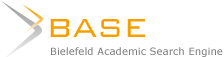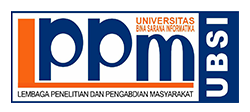### THE INFLUENCE OF ROLE PLAY ON STUDENTS’ ABILITY IN SPEAKING ENGLISH AT THE FIRST GRADE OF SMK USWATUN HASANAH JAKARTA

Ria Yuliasari

#### Sari

The objective of this research is to find out whether there is an influence of Role Play on students’ ability in speaking English. The population in this research was the entire first grade students of SMK USWATUN HASANAH JAKARTA, consist of 60 students divided into 2 classes and the sampling method is Random Sampling, the sample consist of one class with 30 students. The result of Role Play and instrument of the test of speaking consist of 30 items that have been analyzed through validity test using construct validity, reliability test using KR-21, discrimination index and difficulty index analysis. The normality of X variable r-count is = 0.111 which less than r-table is = 0.161, the normality of Y variable r-count is = 0.066 which less than r-table is = 0.161. So that the hypothesis Ho is accepted, it means that the population of the result is normal. The result of data analysis through ANOVA table got r-count is = 5.96 which is more than r-table is = 4.01. The reliability of X variable r-count is = 0.848 which is more than r-table is = 0.361, the reliability Y variable r-count is = 0.885 which is more than r-table is = 0.361. The normality of X variable rcount
is = 0.111 which less than r-table is = 0.161, the normality of Y variable r-count is = 0.066 which less than r-table is = 0.161. So, that the hypothesis Ha is accepted and Ho is rejected. Therefore, it can be concluded that there is an influence of Role Play on students’ ability in speaking English.

#### Teks Lengkap:

PDF (English)

DOI: https://doi.org/10.31294/w.v6i1.3826

### Index by:######### Jl. Kramat Raya No.98, Kwitang, Kec. Senen, Kota Jakarta Pusat, DKI Jakarta 10450